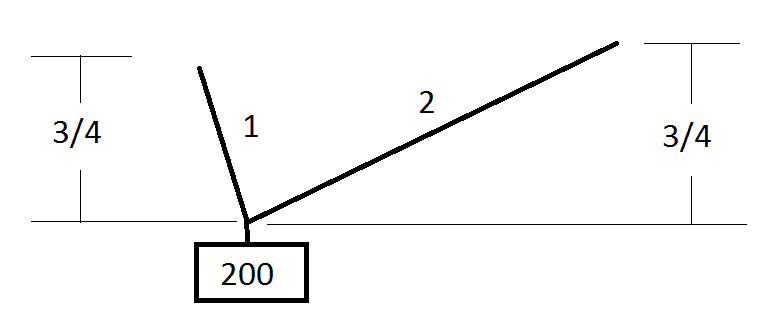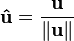# Vector Math to Find Tension

ParoXsitiC

## Homework Statement

Find the tension on the strings using vectors.This is such an easy problem for me when I was in my physics class, we just summed up the forces in the x-direction set it to the force of the weight in the x, and did the same for y.

When introduced into vectors in calc 3 I realized hey it's basically the same thing. However the notion of a normalize vector really threw me off. Apparently a vector isn't correct if its magnitude isn't 1, if that's the case you have to divide it by it's magnitude

## Homework Equations-R = T1 + T2

## The Attempt at a Solution

I found the x components of the triangles using the pythagorean theorem.

√x2+(3/4)2 = 2
x = ± 1/4 √55

√x2+(3/4)2 = 1
x = ± 1/4 √7

I took the negative answer of 1/4 √7, because I am assuming that right is positive so the x-component is left, thus negative.

-200<0,-1> = T1 * 2<1/4√55, 3/4> + T2 * 1<-1/4√7, 3/4>

At this point I am not sure, it's my understanding from physics that you don't distribute the magnitudes into the vectors, only if they are in terms of cosine and sin. So I decided that magnitude of 2 was somehow already incorporated into 1/4√55.

<0,200> = T1 <1/4√55, 3/4> + T2 <-1/4√7, 3/4>

0 = T1 * 1/4√55 - T2 * 1/4√7
200 = T1 * 3/4 + T2 * 3/4

Solving system of equations:

T1 = 70.11898262
T2 = 196.5476841

It seems right, and this is how I would done it in physics but the notion of "magnitude" rather it applies to the tension or the length of the string in this case is really throwing me off, and if I have to normalize the vector by dividing it by it's magnitude, and which magnitude? tension or length? I believe learning vectors in physics first messes up my calculus understanding, or my teacher confused me.

Muphrid

## Homework Statement

Find the tension on the strings using vectors.This is such an easy problem for me when I was in my physics class, we just summed up the forces in the x-direction set it to the force of the weight in the x, and did the same for y.

When introduced into vectors in calc 3 I realized hey it's basically the same thing. However the notion of a normalize vector really threw me off. Apparently a vector isn't correct if its magnitude isn't 1, if that's the case you have to divide it by it's magnitude

There is no such notion of a "correct" vector. What's going on is that you know the tension must act in the same direction as the length of the ropes, but you don't know how much tension there is.

Each rope has a length $\ell$ (a vector). Each rope has a tension $T$ also a vector, but since the tension must be in the same direction as the length, then you know that the tension is $T = |T| \ell/|\ell| = |T| \hat \ell$. That's all we're doing. The unit vector $\hat \ell$ is the same as we'd get if we used sines and cosines directly.

So, for each rope, there are two vectors--a length vector and a tension vector. They both have the same direction, and you're using this information to simplify the problem down from solving for both components of tension to just the tension's magnitude.

ParoXsitiC
Doing it the cosine and sin way (on maple)

http://i.minus.com/iJrTwOn9Y5eqt.png [Broken]

As you can see; if I was to normalize the vector , the magnitudes (lengths of rope) would be divided I would have got the same answer when I divided 2 into both 1/4 sqrt(55) and 3/4.

I am guessing? that you should normalize the vector

Last edited by a moderator:
ParoXsitiC
There is no such notion of a "correct" vector. What's going on is that you know the tension must act in the same direction as the length of the ropes, but you don't know how much tension there is.

Each rope has a length $\ell$ (a vector). Each rope has a tension $T$ also a vector, but since the tension must be in the same direction as the length, then you know that the tension is $T = |T| \ell/|\ell| = |T| \hat \ell$. That's all we're doing. The unit vector $\hat \ell$ is the same as we'd get if we used sines and cosines directly.

So, for each rope, there are two vectors--a length vector and a tension vector. They both have the same direction, and you're using this information to simplify the problem down from solving for both components of tension to just the tension's magnitude.

OK I understand, we are really finding the tension vector but it's in the same direction as the ropes vector so we can just use the ropes vector. However since their is no "correct" way to have a vector, which one of these is right:

Distributing the magnitude:
2<1/4 sqrt(55), 3/4> = <1/2 sqrt(55), 3/2>

Normalizing:

1/2<1/4 sqrt(55), 3/4> = <1/8 sqrt(55), 3/8>

Ignoring the magnitude:

<1/4 sqrt(55), 3/4>

Last edited:
Muphrid
Yes, when I say "direction," I mean a unit (normalized) vector which could be expressed totally in terms of sines and cosines. The tension and the length have the same direction--the same unit vector--but different magnitudes.

Question: why did you do sine of inverse sine?

ParoXsitiC
Yes, when I say "direction," I mean a unit (normalized) vector which could be expressed totally in terms of sines and cosines. The tension and the length have the same direction--the same unit vector--but different magnitudes.

Question: why did you do sine of inverse sine?

Ok thanks, just to show my work that the inverse sin was used in both spots.